# Compressed Sensing of Images¶

Important: Please read the installation page for details about how to install the toolboxes. $\newcommand{\dotp}{\langle #1, #2 \rangle}$ $\newcommand{\enscond}{\lbrace #1, #2 \rbrace}$ $\newcommand{\pd}{ \frac{ \partial #1}{\partial #2} }$ $\newcommand{\umin}{\underset{#1}{\min}\;}$ $\newcommand{\umax}{\underset{#1}{\max}\;}$ $\newcommand{\umin}{\underset{#1}{\min}\;}$ $\newcommand{\uargmin}{\underset{#1}{argmin}\;}$ $\newcommand{\norm}{\|#1\|}$ $\newcommand{\abs}{\left|#1\right|}$ $\newcommand{\choice}{ \left\{ \begin{array}{l} #1 \end{array} \right. }$ $\newcommand{\pa}{\left(#1\right)}$ $\newcommand{\diag}{{diag}\left( #1 \right)}$ $\newcommand{\qandq}{\quad\text{and}\quad}$ $\newcommand{\qwhereq}{\quad\text{where}\quad}$ $\newcommand{\qifq}{ \quad \text{if} \quad }$ $\newcommand{\qarrq}{ \quad \Longrightarrow \quad }$ $\newcommand{\ZZ}{\mathbb{Z}}$ $\newcommand{\CC}{\mathbb{C}}$ $\newcommand{\RR}{\mathbb{R}}$ $\newcommand{\EE}{\mathbb{E}}$ $\newcommand{\Zz}{\mathcal{Z}}$ $\newcommand{\Ww}{\mathcal{W}}$ $\newcommand{\Vv}{\mathcal{V}}$ $\newcommand{\Nn}{\mathcal{N}}$ $\newcommand{\NN}{\mathcal{N}}$ $\newcommand{\Hh}{\mathcal{H}}$ $\newcommand{\Bb}{\mathcal{B}}$ $\newcommand{\Ee}{\mathcal{E}}$ $\newcommand{\Cc}{\mathcal{C}}$ $\newcommand{\Gg}{\mathcal{G}}$ $\newcommand{\Ss}{\mathcal{S}}$ $\newcommand{\Pp}{\mathcal{P}}$ $\newcommand{\Ff}{\mathcal{F}}$ $\newcommand{\Xx}{\mathcal{X}}$ $\newcommand{\Mm}{\mathcal{M}}$ $\newcommand{\Ii}{\mathcal{I}}$ $\newcommand{\Dd}{\mathcal{D}}$ $\newcommand{\Ll}{\mathcal{L}}$ $\newcommand{\Tt}{\mathcal{T}}$ $\newcommand{\si}{\sigma}$ $\newcommand{\al}{\alpha}$ $\newcommand{\la}{\lambda}$ $\newcommand{\ga}{\gamma}$ $\newcommand{\Ga}{\Gamma}$ $\newcommand{\La}{\Lambda}$ $\newcommand{\si}{\sigma}$ $\newcommand{\Si}{\Sigma}$ $\newcommand{\be}{\beta}$ $\newcommand{\de}{\delta}$ $\newcommand{\De}{\Delta}$ $\newcommand{\phi}{\varphi}$ $\newcommand{\th}{\theta}$ $\newcommand{\om}{\omega}$ $\newcommand{\Om}{\Omega}$

This tour explores compressed sensing of natural images, using different sparsity priors over a wavelet basis.

In :
addpath('toolbox_signal')


## Low Pass Linear Measures¶

We first make use of $P$ low pass linear measurements to remove the low frequency content of the image.

Natural images are not only sparse over a wavelet domain. They also exhibit a fast decay of the coefficient through the scale. The coarse (low pass) wavelets caries much of the image energy. It thus make sense to measure directly the low pass coefficients.

We load an image $f \in \RR^{n^2}$ of $n \times n$ pixels.

In :
name = 'boat';
n = 256;
f = rescale(f);


Shortcuts for the wavelet transform $\{\dotp{f}{\psi_m}\}_m$. We only compute up to a scale $J$ so that only $k_0$ sub-bands are transformed.

In :
k0 = 2;
J = log2(n)-k0;
Wav  = @(f)perform_wavelet_transf(f,J,+1);
WavI = @(x)perform_wavelet_transf(x,J,-1);


Compute the wavelet transform.

In :
fw = Wav(f);


Display the coefficients.

In :
clf;
plot_wavelet(fw, J);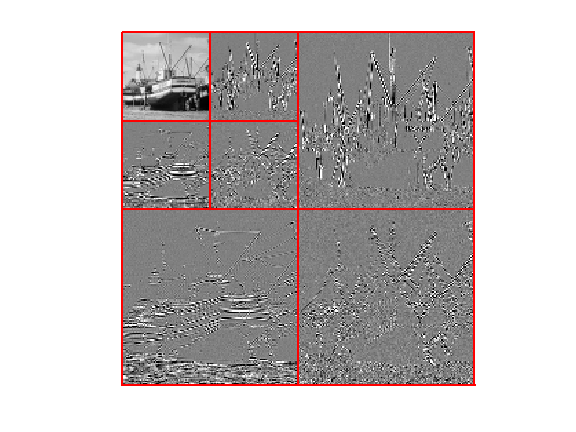Exercise 1

Compute an approximation |fLow| using the $P=2^{2J}=(n/k_0)^2$ low pass coefficients.

In :
exo1()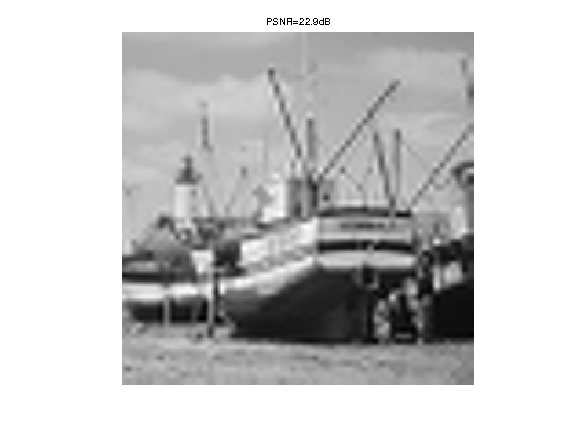In :
%% Insert your code here.


## Randomized Orthogonal Measurements¶

We consider a compressed sensing operator that corresponds to randomized orthogonal projections.

Extract the high pass wavelet coefficients, $x_0 = \{ \dotp{f}{\psi_m} \}_{m \in I_0}$.

In :
A = ones(n,n); A(1:2^J,1:2^J) = 0;
I0 = find(A==1);
x0 = fw(I0);


Number of coefficients.

In :
N = length(x0);


Number $P_0 = 2^{2J}=(n/k_0)^2$ of low pass measurements.

In :
P0 = (n/2^k0)^2;


Number of CS measurements.

In :
P = 4 * P0;


Generate random permutation operators $S_1,S_2 : \RR^N \rightarrow \RR^N$ so that $S_k(x)_i = x_{\sigma_k(i)}$ where $\sigma_k \in \Sigma_N$ is a random permutation of $\{1,\ldots,N\}$.

In :
sigma1 = randperm(N)';
sigma2 = randperm(N)';
S1 = @(x)x(sigma1);
S2 = @(x)x(sigma2);


The adjoint (and also inverse) operators $S_1^*,S_2^*$ (denoted |S1S,S2S|) corresponds to the inverse permutation $\sigma_k^*$ such that $\sigma_k^* \circ \sigma_k(i)=i$.

In :
sigma1S = 1:N; sigma1S(sigma1) = 1:N;
sigma2S = 1:N; sigma2S(sigma2) = 1:N;
S1S = @(x)x(sigma1S);
S2S = @(x)x(sigma2S);


We consider a CS operator $\Phi : \RR^N \rightarrow \RR^P$ that corresponds to a projection on randomized atoms $$(\Phi x)_i = \dotp{x}{ \phi_{\sigma_2(i)}}$$ where $\phi_i$ is a scrambled orthogonal basis $$\phi_i(x) = c_i( \sigma_1(x) )$$ where $\{ c_i \}_i$ is the orthogonal DCT basis.

This can be rewritten in compact operator form as $$\Phi x = ( S_2 \circ C \circ S_1 (x) ) \downarrow_P$$ where $S_1,S_2$ are the permutation operators, and $\downarrow_P$ selects the $P$ first entries of a vector.

In :
downarrow = @(x)x(1:P);
Phi = @(x)downarrow(S2(dct(S1(x))));


The adjoint operator is $$\Phi^* x = S_1^* \circ C^* \circ S_2^* (x\uparrow_P)$$ where $\uparrow_P$ append $N-P$ zeros at the end of a vector, and $C^*$ is the inverse DCT transform.

In :
uparrow = @(x)[x; zeros(N-P,1)];
PhiS = @(x)S1S(idct(S2S(uparrow(x))));


Perform the CS (noiseless) measurements.

In :
y = Phi(x0);


Exercise 2

Reconstruct an image using the pseudo inverse coefficients $\Phi^+ y = \Phi^* y$.

In :
exo2()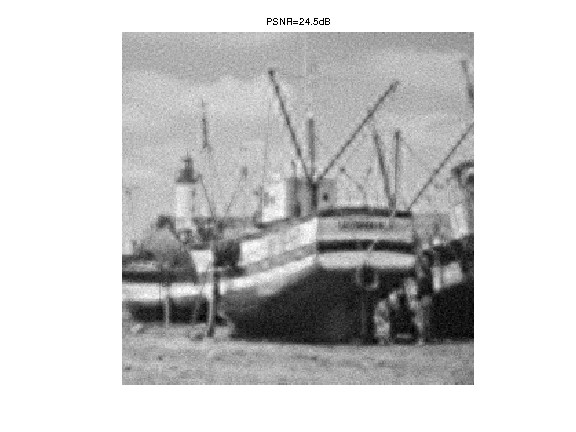In :
%% Insert your code here.


## Compressed Sensing Recovery using Douglas Rachford Scheme¶

We consider the minimum $\ell^1$ recovery from the measurements $y = \Phi x_0 \in \RR^P$ $$\umin{\Phi x = y} \normu{x}.$$ This can be written as $$\umin{ x } F(x) + G(x) \qwhereq \choice{ F(x) = i_{\Cc}(x), \\ G(x) = \normu{x}. }$$ where $\Cc = \enscond{x}{\Phi x =y}$.

One can solve this problem using the Douglas-Rachford iterations $$\tilde x_{k+1} = \pa{1-\frac{\mu}{2}} \tilde x_k + \frac{\mu}{2} \text{rPox}_{\gamma G}( \text{rProx}_{\gamma F}(\tilde x_k) ) \qandq x_{k+1} = \text{Prox}_{\gamma F}(\tilde x_{k+1},)$$

We have use the following definition for the proximal and reversed-proximal mappings: $$\text{rProx}_{\gamma F}(x) = 2\text{Prox}_{\gamma F}(x)-x$$ $$\text{Prox}_{\gamma F}(x) = \uargmin{y} \frac{1}{2}\norm{x-y}^2 + \ga F(y).$$

One can show that for any value of $\gamma>0$, any $0 < \mu < 2$, and any $\tilde x_0$, $x_k \rightarrow x^\star$ which is a solution of the minimization of $F+G$.

Exercise 3

Implement the proximal and reversed-proximal mappings of $F$ (the orthogonal projector on $\Cc$ and $G$ (soft thresholding). In Matlab, use inline function with the |@| operator.

In :
exo3()

In :
%% Insert your code here.


Value for the $0 < \mu < 2$ and $\gamma>0$ parameters. You can use other values, this might speed up the convergence.

In :
mu = 1;
gamma = 1;


Exercise 4

Implement the DR iterative algorithm. Keep track of the evolution of the $\ell^1$ norm $G(x_k)$.

In :
exo4()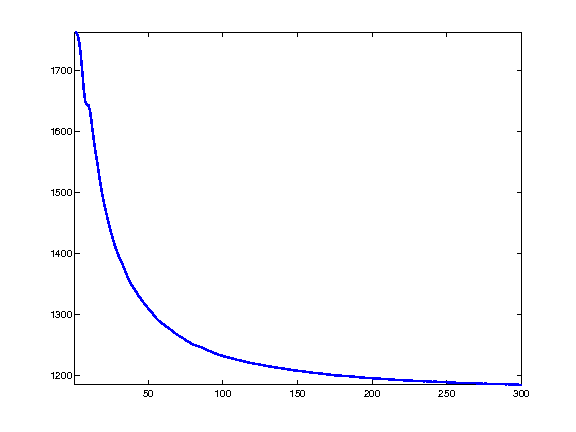In :
%% Insert your code here.


Exercise 5

Display the image reconstructed using the $P_0$ linear and $P$ CS measurements. The total number of used measurements is thus $P+P_0$.

In :
exo5()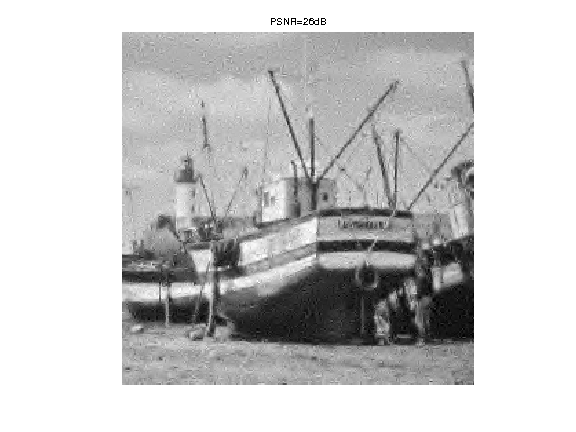In :
%% Insert your code here.


## Compressed Sensing Reconstruction using Block Sparsity¶

In order to enhance the CS reconstruction, it is possible to use more advanced priors than plain $\ell^1$.

One can for instance use a block $\ell^1$ norm $$G(x) = \sum_i \norm{x_{B_i}}$$ where $(B_i)_i$ is a disjoint segmentation of the index set $\{1,\ldots,N\}$, where $x_{B} = \{ x_i \}_{i \in B} \in \RR^{|B|}$ extracts the coefficients within $B$, and $\norm{x_B}$ is the $\ell^2$ norm.

The proximal operator of this block $\ell^1$ norm is a block thresholding $$\forall \, m \in B_i, \quad \text{Prox}_{\ga G}(x)_i = \max(0, 1-\ga/\norm{x_{B_i}}) x_i.$$

We use uniform blocks of size $w \times w$.

In :
w = 4;


Blocks position and offset in the image domain.

In :
v = 1:w:n;
dv = 0:w-1;
[dX,dY,X,Y] = ndgrid(dv,dv,v,v);
q = size(X,3);
dX = reshape(dX, [w*w q*q]);
dY = reshape(dY, [w*w q*q]);
X = reshape(X, [w*w q*q]);
Y = reshape(Y, [w*w q*q]);


Remove the block which fails outside the image.

In :
I = find( sum(X+dX>n | Y+dY>n)  );
X(:,I) = [];
Y(:,I) = [];
dX(:,I) = [];
dY(:,I) = [];


Compute the indexes of the block in $\{1,\ldots,N\}$, i.e. not in image space but over the CS coefficients space.

In :
U = zeros(n,n);
U(I0) = 1:N;
Ind = X+dX + (Y+dY-1)*n;
I = U(Ind);


Remove the indexes that corresponds to low pass wavelet coefficients.

In :
I(:,sum(I==0)>0) = [];


A block is defined as $B_i = \{ I_{k,i} \}_{k=1}^{w^2}$. Define the energy.

In :
G = @(x)sum( sqrt(sum(x(I).^2)) );


Just for check : display in coefficient space the block structure.

In :
[A,tmp] = meshgrid( randperm(size(I,2)) , ones(w*w,1));
x = zeros(N,1); x(I) = A;
Z = zeros(n,n); Z(I0) = x;
clf;
imageplot(Z);
colormap jet(256);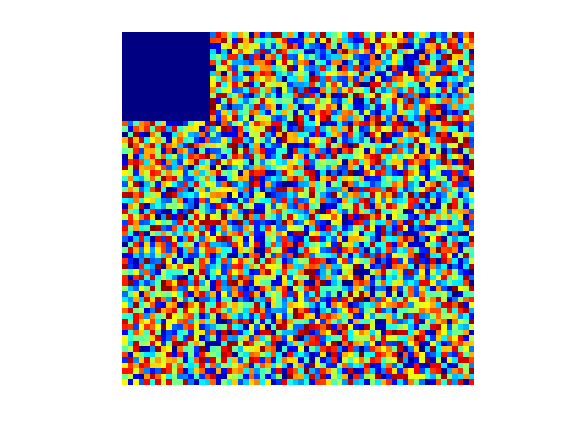Exercise 6

define the proximal operator $\text{Prox}_{\ga G}$ of $G$, and its reversed proximal mapping.

In :
exo6()

In :
%% Insert your code here.


Exercise 7

Implement the DR iterative algorithm. Keep track of the evolution of $G(x_k)$.

In :
exo7()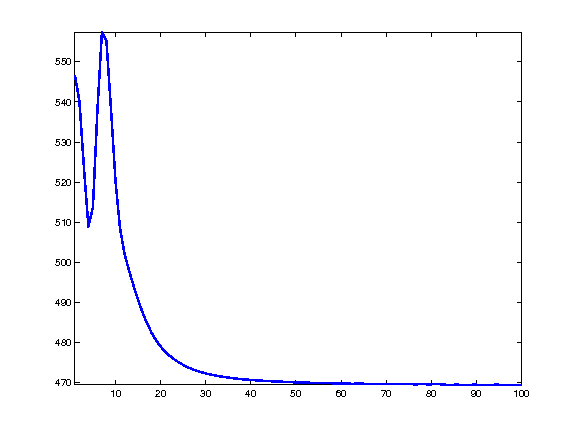In :
%% Insert your code here.


Exercise 8

Display the image reconstructed using the $P_0$ linear and $P$ CS measurements.

In :
exo8()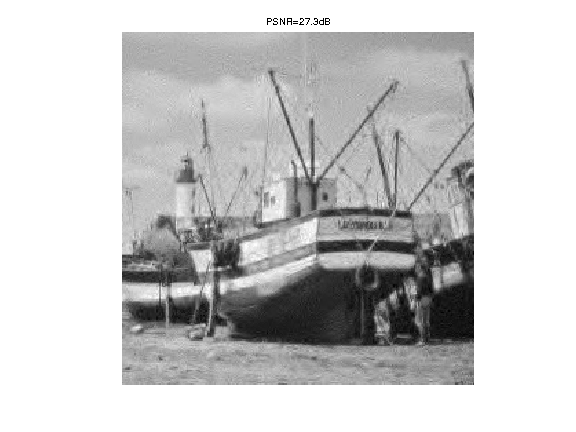In :
%% Insert your code here.